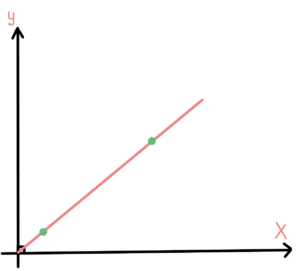# Linear search in c#### Linear search implementation in c#

Linear search, also known as sequential search, is a simple algorithm used to find a specific value in an array or list. It works by iterating through each element of the array or list and comparing it to the target value. If the target value is found, the index of the element is returned. If the target value is not found, the algorithm will return -1. So let’s take a look at linear search in c#.

In C#, the linear search can be easily implemented using a for loop. Here is an example of how to implement a linear search algorithm in C#:

This example creates an array of integers and searches for the number 5. The LinearSearch method takes two parameters, an array of integers and the target value. The method iterates through the array using a for loop, and compares each element to the target value. If the element is equal to the target value, the index of the element is returned. If the target value is not found, the method will return -1. The output of this program will be “The number 5 is at index 4”, which is the index of the number 5 in the array.

### The time complexity

Linear search has a time complexity of O(n), which means that the time it takes to find a specific value increases linearly with the size of the array or list. This makes linear search less efficient than other algorithms, such as binary search, when searching large arrays or lists.

Below you can see the graph that shows how the complexity grows along with the data:### Pros and cons of linear search

Pros:

• Simple to understand and implement: Linear search is a basic algorithm that is easy to understand and implement, making it suitable for beginners.
• No need to sort the data: Linear search does not require the data to be sorted, which makes it more versatile than other search algorithms, such as binary search.
• Can be used with any data type: Linear search can be used with any data type, including arrays and lists of integers, strings, and custom objects.

Cons:

• The time complexity of O(n).
• Inefficient for large datasets: Because linear search has a time complexity of O(n), it becomes less efficient when searching large datasets. This is because it has to iterate through every item in the dataset in order to find the target value.
• Not suitable for large-scale or real-time applications: Due to its inefficiency for large datasets, linear search is not suitable for large-scale or real-time applications where performance is critical.

### Summary

In summary, linear search is a simple algorithm used to find a specific value in an array or list. It can be easily implemented in C# using a for loop or a foreach loop. While linear search is less efficient than other algorithms when searching large arrays or lists, it’s easy to implement and understand.

If you would find a more efficient search, read about binary search.